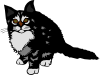Click here for the Problem Extension Worksheet version of the Problems of the Week.
Click here for an MS Word version of the Problems of the Week.
Click here for the Canadian Problem Extension Worksheet version of the Problems of the Week.
Click here for a Canadian MS Word version of the Problems of the Week.Lower Elementary:
Question: Zachary sold books at a yard sale for 15¢ each and toys for \$1.00 each. If he sells 6 books and 3 toys, how much money does Zachary make altogether?
Answer: Zachary makes \$3.90.
Solution: Zachary earned 15¢, 6 times for the books. That’s 15, 30, 45, 60, 75, 90¢ for all 6 of the books. He earned \$1.00, 3 times for the toys. That’s \$3.00 for all 3 of the toys. So, altogether, Zachary earned \$0.90 + \$3.00 = \$3.90.Upper Elementary:
Question: Eight students are performing songs for their music class recital. Each song is 5 minutes long. If each music student performs 1 song and all 8 students must be done performing by 9:15 PM, then what is the latest time they can start the recital?
Answer: The latest they can start is 8:35 PM.
Solution: The amount of time it takes for all of the students to finish their songs is 8 × 5 = 40 minutes. Since they have to finish the recital by 9:15 PM, we count back 40 minutes before 9:15 PM to 8:35 PM.Middle School:
Question: A 9-foot tree casts a 6-foot shadow. A songbird casts a 2-inch shadow. A squirrel is twice as tall as the songbird. How tall is the squirrel?
Answer: The squirrel is 6 inches tall.
Solution: The length of the tree’s shadow is two-thirds the height of the tree. So, the length of the songbird’s shadow is two-thirds its height; 2 inches is two-thirds of 3 inches, so the songbird must be 3 inches tall. That means that the squirrel is 6 inches tall because 6 inches is twice as tall as 3 inches.Algebra and Up:
Question: The profit earned by a cat-sitting company, measured in thousands of dollars, is modeled by the function f(t) = t2 – 8t + 16, wherein t is measured in months and t = 1 is January. During which month does the cat-sitting company earn the least amount of money? How much do they earn?
Answer: They earned \$0.00 in April.
Solution: This function is a parabola that opens upward, so the lowest value is at the vertex. To find the month, we find –b/2a = –(–8)/(2 × 1) = 8/2 = 4. So the vertex is at t = 4, which represents the month of April. To find how much they earned, we set t = 4 and solve: 42 – 8(4) + 16 = 16 – 32 + 16 = 0. So, the cat-sitting business earned \$0.00 in April.